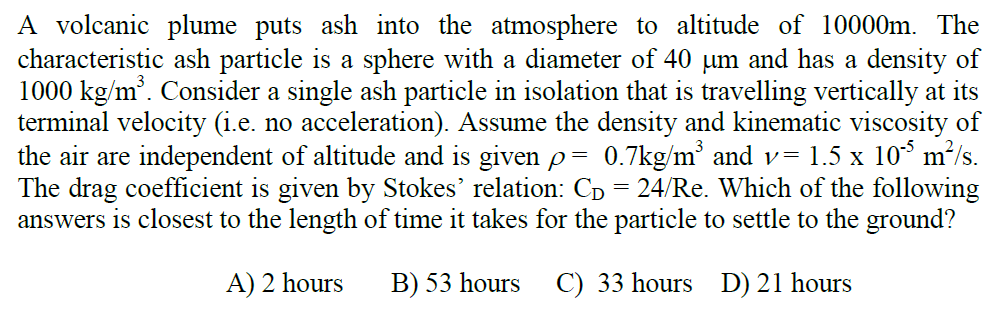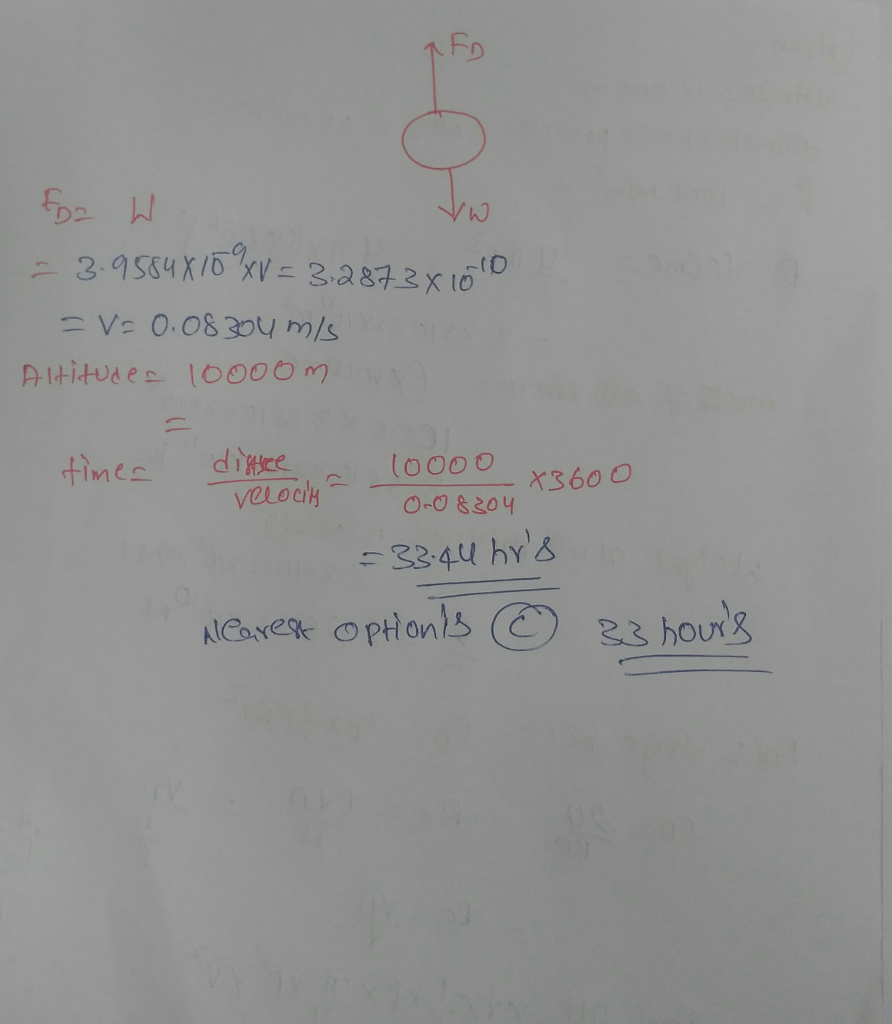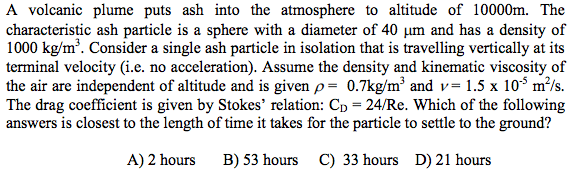Question#### Earn Coins

Coins can be redeemed for fabulous gifts.

Similar Homework Help Questions
• ### A volcanic plume puts ash into the atmosphere to altitude of 10000m. The characteristic ash particle...A volcanic plume puts ash into the atmosphere to altitude of 10000m. The characteristic ash particle is a sphere with a diameter of 40 mum and has a density of 1000 kg/m^3. Consider a single ash particle in isolation that is travelling vertically at its terminal velocity (i.e. no acceleration). Assume the density and kinematic viscosity of the air are independent of altitude and is given rho = 0.7 kg/m^3 and nu = 1.5 times 10^-5 m^2/s. The drag coefficient...